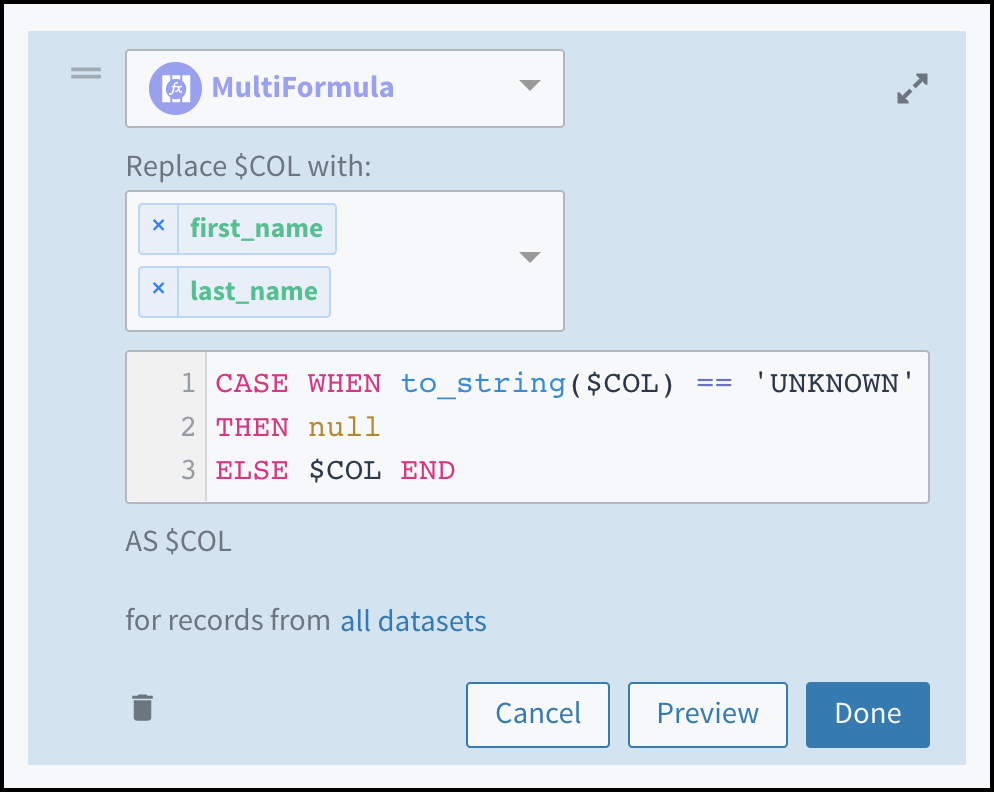User GuidesAPI ReferenceRelease Notes

# Using the MultiFormula Option

The MultiFormula option applies a single expression to multiple attributes.

You use the MultiFormula option to apply the same transformation expression to one or more columns. This option is typically used to apply data cleaning or standardization logic to multiple columns efficiently.

• You can select any number of input columns. The output of the multiformula populates these columns.
• You write an expression to apply to all of the selected columns by using the substitution `\$COL` where, in a formula, you would insert a column name. For example, instead of writing `upper(company_name)` to transform values in a single column, you use `upper(\$COL)` to transform values in all selected columns.

Examples follow.

# Example: Replace a Value with `null`

This example replaces values that match `'UNKNOWN'` in both the `"first_name"` and `"last_name"` columns with `null`.A multiformula with two columns selected and an expression that changes 'UNKNOWN" to null in those columns.

This multiformula expression is equivalent to writing the following script:

``````SELECT *,
CASE WHEN to_string("first_name") == 'UNKNOWN'
THEN null ELSE "first_name" END AS "first_name",
CASE WHEN to_string("last_name") == 'UNKNOWN'
THEN null ELSE "last_name" END AS "last_name";
``````

# Example: Standardize Date Format

This example applies standard ISO format to the dates in the `"PatientBirthDate"`, `"PatientVisitDate"`, and `"RecordUpdateDate"` columns.

``````SELECT *,